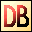DelphiBasicsFormatFunction Rich formatting of numbers and text into a string SysUtils unit
 1 function Format ( Const Formatting : string; Const Data : array of const ) : string; 2 function Format ( Const Formatting : string; Const Data : array of const; FormatSettings : TFormatSettings ) : string;
Description
The Format function provides 'C' like formatting of multiple of simple data types into a string. It provides very precise control over this formatting.

The Formatting parameter defines how the Data array is manipulated into the returned string.

The Formatting string can comprise a mix of ordinary characters (that are passed unchanged to the result string), and data formatting characters. This formatting is best explained by the example code.

In simple terms, each data formatting substring starts with a % and ends with a data type indicator :

 d = Decimal (integer) e = Scientific f = Fixed g = General m = Money n = Number (floating) p = Pointer s = String u = Unsigned decimal x = Hexadecimal

The general format of each formatting substring is as follows:

%[Index:][-][Width][.Precision]Type

where the square brackets refer to optional parameters, and the : . - characters are literals, the first 2 of which are used to identify two of the optional arguments.

Version 2 of this function is for use within threads. You furnish the FormatSettings record before invoking the call. It takes a local copy of global formatting variables that make the routine thread safe.
Notes
Various formatting options, such as DecimalSeparator and CurrencyString are used for some of these formatting options. See the more specific versions of data display commands, such as CurrToStrF for details.
Related commands
 CurrencyDecimals Defines decimal digit count in the Format function CurrencyFormat Defines currency string placement in curr display functions CurrencyString The currency string used in currency display functions CurrToStrF Convert a currency value to a string with formatting DecimalSeparator The character used to display the decimal point FloatToStrF Convert a floating point value to a string with formatting FormatCurr Rich formatting of a currency value into a string FormatDateTime Rich formatting of a TDateTime variable into a string FormatFloat Rich formatting of a floating point number into a string NegCurrFormat Defines negative amount formatting in currency displays ThousandSeparator The character used to display the thousands separator
Author linksDownload this web site as a Windows program.

 Example code : Showing all of the formatting data types var   text : string; begin  // Just 1 data item   ShowMessage(Format('%s', ['Hello']));   // A mix of literal text and a data item   ShowMessage(Format('String = %s', ['Hello']));   ShowMessage('');   // Examples of each of the data types   ShowMessage(Format('Decimal          = %d', [-123]));   ShowMessage(Format('Exponent         = %e', [12345.678]));   ShowMessage(Format('Fixed            = %f', [12345.678]));   ShowMessage(Format('General          = %g', [12345.678]));   ShowMessage(Format('Number           = %n', [12345.678]));   ShowMessage(Format('Money            = %m', [12345.678]));   ShowMessage(Format('Pointer          = %p', [addr(text)]));   ShowMessage(Format('String           = %s', ['Hello']));   ShowMessage(Format('Unsigned decimal = %u', ));   ShowMessage(Format('Hexadecimal      = %x', )); end; Show full unit code Hello   String = Hello      Decimal          = -123   Exponent         = 1.23456780000000E+004   Fixed            = 12345.68   General          = 12345.678   Number           = 12,345,68   Money            = ?12,345.68   Pointer          = 0069FC90   String           = Hello   Unsigned decimal = 123   Hexadecimal      = 8C Example code : Using the index, width and precision values begin  // The width value dictates the output size  // with blank padding to the left  // Note the <> characters are added to show formatting   ShowMessage(Format('Padded decimal    = <%7d>', ));   // With the '-' operator, the data is left justified   ShowMessage(Format('Justified decimal = <%-7d>', ));   // The precision value forces 0 padding to the desired size   ShowMessage(Format('0 padded decimal  = <%.6d>', ));   // A combination of width and precision  // Note that width value precedes the precision value   ShowMessage(Format('Width + precision = <%8.6d>', ));   // The index value allows the next value in the data array  // to be changed   ShowMessage(Format('Reposition after 3 strings = %s %s %s %1:s %s',                      ['Zero', 'One', 'Two', 'Three']));   // One or more of the values may be provided by the  // data array itself. Note that testing has shown that an *  // for the width parameter can yield EConvertError.   ShowMessage(Format('In line           = <%10.4d>', ));   ShowMessage(Format('Part data driven  = <%*.4d>', [10, 1234]));   ShowMessage(Format('Data driven       = <%*.*d>', [10, 4, 1234])); end; Show full unit code Padded decimal    = <   1234>   Justified decimal = <1234   >   0 padded decimal  = <001234>   Width + precision = <  001234>   Reposition after 3 strings = Zero One Two One Two   In line           = <      1234>   Part data driven  = <      1234>   Data driven       = <      1234>
 Delphi Programming © Neil Moffatt 2002 - 2020. All rights reserved.  |  Home Page